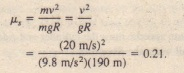# Sample Problem Force And Motion 11

Sample Problem Force And Motion 11

Represents a stock car of mass m =J 600 kg traveling at a constant speed  around a flat, circular track of radius.

SOLUTION: We need to relate , to the circular motion of the car. We start with four Key Ideas, all related to one force on the car.

1. A centripetal force must act on the car if the car is moving around a circular path; the force must be directed horizontally toward the center of the circle.

2. The only horizontal force acting on the car is a frictional force on the tires from the road. So, the required centripetal force is a frictional force.

3. Because the car is not sliding, the frictional force must be a static frictional force.

4. If the car is just on the verge of sliding, the magnitude Is of the frictional force is just equal to the maximum value , where N is the magnitude of the normal force on the car from the track.(a) Acar moves around aflat curved road atconstant speed v.The frictional force t.provides the necessarycentripetalforce along a radialaxis r. (b) A free bodydiagram (not toscale) for the car, inthe vertical planecontaining.

Because the car does not accelerate vertically, the two vertical. forces acting on it  must balance; that is, the magnitude N of the normal force must equal the magnitude mg of the gravitational force. Substituting N = mg into we find,This means that if IL, = 0.21, the car is on the verge of sliding off the track; the car is in no danger of sliding off; and the car will certainly slide off.

Equation 6-24 contains two important lessons for road engineers. First, the value of, (required to prevent sliding) depends on the square of v. Much more friction is required when the turning speed is increased. You may have noted this effect if you have ever  a flat turn too fast and suddenly felt the tires slip. Second, the mass m dropped out in our derivation of Thus,  holds for a vehicle of any mass, from a kindly car to a bicycle to a heavy truck.

CHECKPOINT 6:Suppose the car is on the Car verge of sliding when the radius of the circle is double the car’s speed.